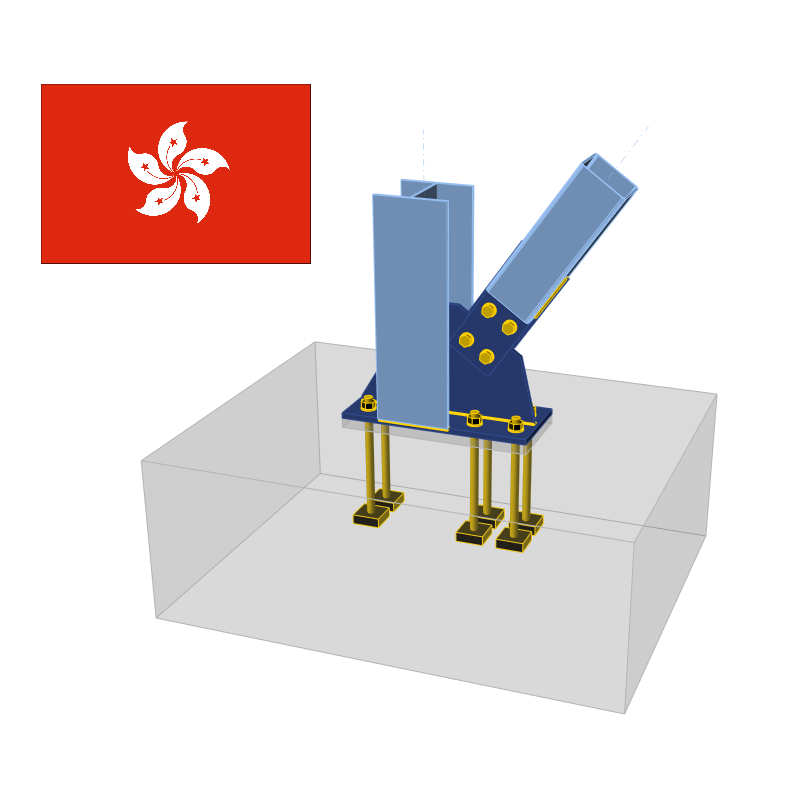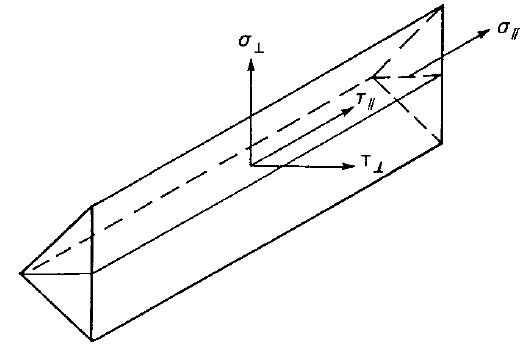### Alegeți limba# Check of components according to HKG (Hong Kong Code of Practice)

SupportCenter.LocalizedArticle
Bolts, preloaded bolts, and welds are checked according to Code of Practice for the Structural Use of Steel 2011 (CoP – SUoS). Concrete in bearing is designed according to Code of Practice for Structural Use of Concrete 2013 (CoP – SUoC).
$$Design check of plates according to Hong Kong Standard Plates in CBFEM are modeled by shell finite elements. The plastic strain must not exceed the limit. The resulting equivalent stress (HMH, von Mises) and plastic strain are calculated on plates. When the design yield strength, $$p_y$$ (Cl. 3.1.2), on the bilinear material diagram is reached, the check of the equivalent plastic strain is performed. The limit value of 5 % is suggested in Eurocode (EN 1993-1-5 App. C, Par. C8, Note 1). This value can be modified in Code setup but verification studies were made for this recommended value. Plate element is divided into 5 layers and elastic/plastic behavior is investigated in each of them. The program shows the worst result of all of them.Stress may be a little bit higher than design yield strength. The reason is the slight inclination of the plastic branch of the stress-strain diagram, which is used in the analysis to improve the stability of the calculation.$p_y = \min \left \{ \frac{Y_s}{\gamma_{m1}}, \frac{U_s}{\gamma_{m2}} \right \}$where: $$p_y$$ – design yield strength $$Y_s$$ – characteristic yield strength $$U_s$$ – minimum tensile strength $$\gamma_{m1}$$ – material factor (Table 4.1); default value $$\gamma_{m1} = 1$$ editable in Code setup $$\gamma_{m2}$$ – material factor (Table 4.1); default value $$\gamma_{m2} = 1.2$$ editable in Code setup Check of welds according to Hong Kong Code Welds are designed according to Cl. 9.2 – Simplified method. Butt weldsFull penetration butt welds are expected and their resistance is considered equal to that of the parent metal – Cl. 9.2.5.2.1.Fillet weldsFillet welds are designed by Simplified method according to Cl. 9.2.5.1.6. $f_w \le p_w$ $$f_w = \sqrt{\sigma_\perp ^2 + \tau_\perp ^2 + \tau_\parallel ^2}$$ – vector sum of stresses in weld throat in all directions $$p_w$$ – design strength of fillet weld determined according to Table 9.2a and 9.2b; for cases not covered by Tables 9.2a and 9.2b: $$p_w = \min \{0.5 U_e, 0.55 U_s\}$$ – for EN electrode used with EN steel $$p_w = 0.38 \min \{U_e, U_s\}$$ – for other cases $$U_e$$ – minimum tensile strength of electrode $$U_s$$ – minimum tensile strengthThe effective length of the fillet weld is reduced by $$2\cdot s$$ according to Cl. 9.2.5.1.3, where $$s$$ is the fillet weld leg size assumed equal to $$a\cdot \sqrt{2}$$. Electrode Steel grade354250 S 275220220220 S 355220250250 S 460220250280 Steel gradeElectrodeDesign strength Q235E43160 Q345E50200 Q390, Q420E55220 Tables 9.2a and 9.2b: Design strengths $$p_w$$ [MPa] Welding electrodeMinimum tensile strength $$U_e$$ [MPa] 35440 42500 50560 E43421.1 E50526.3 E55578.9 Default minimal tensile strength of electrode $$U_e$$ [MPa]The weld diagrams show stress according to the following formula:$\sigma = \sqrt{\sigma_{\perp}^2 + \tau_{\perp}^2 + 3 \tau_{\parallel}^2 }$ Check of bolts according to Hong Kong Code Bolts are checked for shear, bearing, tension capacity and for combined tension and shear. Bolts in tensionBolt resistance in tension is checked according to Cl. 9.3.7.1 as:$P_t = A_s \cdot p_t$where: $$A_s$$ – tensile stress area $$p_t$$ – tension strength obtained from Table 9.8 Prying forces are taken into account by finite element analysis.Bolts in shearShear capacity of bolts is taken according to Cl. 9.3.6.1.1 as:$P_s = p_s \cdot A_s$where: $$p_s$$ – design shear strength obtained from Table 9.5 $$A_s$$ – effective shear area; $$A_s = A_t$$ if threads are intercepted by shear plane, $$A_s$$ is taken as the cross sectional area of the shank otherwise $$A_t$$ – tensile area According to Cl. 9.3.6.1.6, when a bolt passes through packing with thickness $$t_{pa}$$ greater than one-third of the nominal diameter $$d$$, its shear capacity $$P_s$$ should be reduced by multiplying a reduction factor $$\beta_p$$ obtained from:$\beta_p = \frac{9d}{8d+3t_{pa}} \le 1$Bolts in combined tension and shearCombined tension and shear is checked according to Cl. 9.3.8.1 as:$\frac{F_s}{P_s} + \frac{F_{tot}}{P_t} \le 1.4$where: $$F_s$$ – shear force in a bolt $$P_s$$ – shear resistance of a bolt $$F_{tot}$$ – total applied tension in the bolt including the prying force $$P_t$$ – tensile resistance of a bolt Bolts in bearingBearing capacity of bolts is taken according to Cl. 9.3.6.1.2 as:$P_{bb} = d \cdot t_p \cdot p_{bb}$where: $$d$$ – nominal diameter of the bolt $$t_p$$ – thickness of the connected plate $$p_{bb}$$ – bearing strength of the bolt obtained from Table 9.6 Each plate is checked separately and the worst result is shown.Bearing capacity of connected parts is taken according to Cl. 9.3.6.1.3 as the minimum of the following:$P_{bs} = k_{bs} \cdot d \cdot t_p \cdot p_{bs}$$P_{bs} = 0.5 \cdot k_{bs} \cdot e \cdot t_p \cdot p_{bs}$$P_{bs} = 1.5 \cdot l_c \cdot t_p \cdot U_s \le 2.0 \cdot d \cdot t_p \cdot U_b$where: $$k_{bs}$$ – hole coefficient taken as for standard holes $$k_{bs} = 1.0$$ for oversized and short slotted holes $$k_{bs} = 0.7$$ for long slotted holes $$k_{bs} = 0.5$$ $$d$$ – nominal bolt diameter $$t_p$$ – connected plate thickness $$p_{bs}$$ – bearing strength of connected parts for steel of grade S275, $$p_{bs} = 460$$ MPa for steel of grade S355, $$p_{bs} = 550$$ MPa for steel of grade S460, $$p_{bs} = 670$$ MPa for steel of other grades, $$p_{bs} = 0.67 (U_s+Y_s)$$ $$e$$ – edge distance in the direction of the shear force measured from the centreline of the bolt $$l_c$$ – net distance between the bearing edge of the holes and the near edge of adjacent hole in the same direction of load transfer $$U_s$$ – minimum tensile strength of connected plate $$Y_s$$ – characteristic yield strength of connected plate $$U_b$$ – specified minimum tensile strength of bolt Check of preloaded bolts according to Hong Kong Code Preloaded bolts are checked for shear slip capacity and combination of tension and shear. Shear capacityShear capacity of preloaded bolts is determined according to Cl. 9.3.6.2 as:$P_{SL} = 0.9 \cdot K_s \cdot \mu \cdot P_0$ where: $$K_s$$ – hole coefficient taken as for standard holes $$K_s = 1.0$$ for oversized holes $$K_s = 0.85$$ for slotted holes $$K_s = 0.7$$ $$\mu$$ – slip factor between connected parts from Table 9.7; editable in Code setup $$P_0$$ – minimum proof loads of bolts specified in relevant international or local standards Combined tension and shearCombined tension and shear is checked according to Cl. 9.3.8.2 as:$\frac{F_s}{P_{SL}}+\frac{F_{tot}}{0.9\cdot P_0} \le 1.0$where: $$F_s$$ – shear force in a bolt $$P_{SL}$$ – slip resistance of a preloaded bolt $$F_{tot}$$ – total applied tension in the bolt including the prying force $$P_0$$ – specified minimum proof load of a preloaded bolt Check of concrete block according to HKG The average stress on a base plate area in contact with concrete is checked against the concrete bearing resistance. Concrete in bearingConcrete in bearing is checked according to CoP – SUoS – Cl. 9.4.1 as:$\sigma \le w$where: $$\sigma$$ – average compressive stress at effective area $$A_{eff}$$ which is the intersection of two areas: $$A_{CM}$$ – effective area determined according to Cl. 9.4.1 for pure compression $$A_{FEM}$$ – area under the base plate in contact with concrete determined by FEA $$w = 0.6 f_{cu}$$ – concrete compressive resistance against concentrated load $$f_{cu}$$ – concrete minimum characteristic compressive strength Effective area $$A_{CM}$$ is the area of steel member including stiffeners welded to the base plate increased by overlap $$c$$:$c = t_p \sqrt{\frac{p_{yp}}{3w}}$where: $$t_p$$ – base plate thickness $$p_{yp}$$ – design yield strength of the base plate The pressure under the compression zone is considered as uniform.Transfer of shearThe shear action at the base plate is assumed to be transferred from the column to the concrete foundation by: Friction between base plate and concrete/grout Shear lug Anchor bolts AnchorsThe tensile forces in anchors include prying forces and are determined by finite element analysis.Anchors are not checked in the software. Detailing of bolts and welds according to Hong Kong Code Detailing of bolt spacing and edge distance and weld minimal size. BoltsBolt minimum spacing is according to Cl. 9.3.1.1: Centre to centre of bolt should be larger than $$2.5 \cdot d$$, where $$d$$ is nominal bolt diameter.Minimum edge distance measured from the centreline of the bolt is according to Table 9.3: Bolt sizeMinimum edge distance [mm] M1218 M1622 M1824 M2026 M2228 M24 and over$$1.25 \cdot d$$ WeldsMinimum leg size of fillet welds is checked according to Table 9.1. Thickness of the thickest part [mm]Minimum leg length [mm]Minimum throat thickness [mm] $$t \le 6$$32.121 $$6 < t \le 13$$53.536 $$13 < t \le 19$$64.243 $$19 > t$$85.657 Capacity design for Hong Kong Code Capacity design is not required by Hong Kong standards. Classification according to stiffness for Hong Kong Code Joints are classified according to their moment stiffness according to Eurocode. Joints are classified according to joint stiffness to: Rigid – joints with insignificant change of original angles between members, Semirigid – joints which are assumed to have the capacity to furnish a dependable and known degree of flexural restraint, Pinned – joints which do not develop bending moments. Joints are classified according to the EN 1993-1-8 – Cl. 5.2.2. Rigid – $$\frac{S_{j,ini} L_b}{E I_b} \ge k_b$$ Semirigid – $$0.5 < \frac{S_{j,ini} L_b}{E I_b} < k_b$$ Pinned – $$\frac{S_{j,ini} L_b}{E I_b} \le 0.5$$ where: Sj,ini – initial stiffness of the joint; the joint stiffness is assumed linear up to the 2/3 of Mj,Rd Lb – theoretical length of the analyzed member; set in member properties E – Young's modulus of elasticity Ib – moment of inertia of the analyzed member kb = 8 for frames where the bracing system reduces the horizontal displacement by at least 80 %; kb = 25 for other frames, provided that in every storey Kb/Kc ≥ 0.1. The value of kb = 25 is used unless the user sets "braced system" in Code setup. Mj,Rd – joint design moment resistance Kb = Ib / Lb Kc = Ic / Lc$$